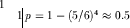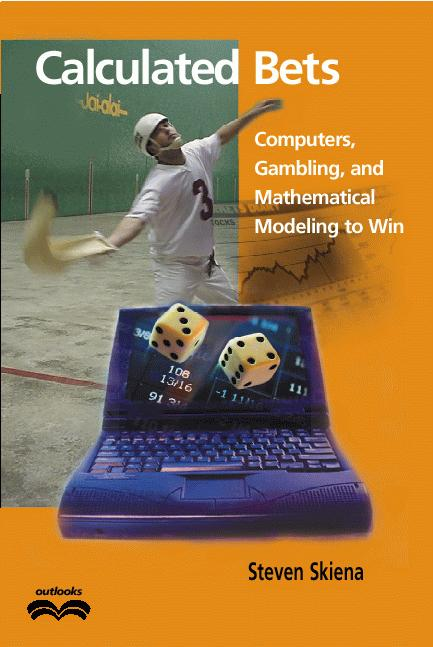Next: Jai-Alai Players and Baseball Up: 6. Is this Bum Previous: 6. Is this Bum

# Probability versus Statistics

Probability and statistics are related areas of mathematics which concern themselves with analyzing the relative frequency of events. Still, there are fundamental differences in the way they see the world:

• Probability deals with predicting the likelihood of future events, while statistics involves the analysis of the frequency of past events.

• Probability is primarily a theoretical branch of mathematics, which studies the consequences of mathematical definitions. Statistics is primarily an applied branch of mathematics, which tries to make sense of observations in the real world.

Both subjects are important, relevant, and useful. But they are different, and understanding the distinction is crucial in properly interpreting the relevance of mathematical evidence. Many a gambler has gone to a cold and lonely grave for failing to make the proper distinction between probability and statistics.

This distinction will perhaps become clearer if we trace the thought process of a mathematician encountering her first craps game:

• If this mathematician were a probabilist, she would see the dice and think ``Six-sided dice? Presumably each face of the dice is equally likely to land face up. Now assuming that each face comes up with probability 1/6, I can figure out what my chances of crapping out are.''

• If instead a statistician wandered by, she would see the dice and think ``Those dice may look OK, but how do I know that they are not loaded? I'll watch a while, and keep track of how often each number comes up. Then I can decide if my observations are consistent with the assumption of equal-probability faces. Once I'm confident enough that the dice are fair, I'll call a probabilist to tell me how to play.''

In summary, probability theory enables us to find the consequences of a given ideal world, while statistical theory enables us to to measure the extent to which our world is ideal.

Modern probability theory emerged from the dice tables of France in 1654. Chevalier de Méré, a French nobleman, wondered whether the player or the house had the advantage in a variation of the following betting game.6.1 In the basic version, the player rolls four dice, and wins provided none of them are a six. The house collects on the even money bet if at least one six appears.

De Méré brought this problem to attention of the French mathematicians Blaise Pascal and Pierre de Fermat, most famous as the source of Fermat's Last Theorem. Together, these men worked out the basics of probability theory, along the way establishing that the house wins the basic version with probability, where the probability p = 0.5 would denote a fair game where the house wins exactly half the time.    The jai-alai world of our Monte Carlo simulation assumes that we decide the outcome of a point between two teams by flipping a suitably biased coin. If this world were reality, our simulation will compute the correct probability of each possible betting outcome. But all players are not created equal, of course. By doing a statistical study of the outcome of all the matches involving a particular player, we can determine an appropriate amount to bias the coin.

But such computations only make sense if our simulated jai-alai world is a model consistent with the real world. John von Neuman once said that ``the valuation of a poker hand can be sheer mathematics.'' We have to reduce our evaluation of a pelotari to sheer mathematics.I hope you have enjoyed this excerpt from Calculated Bets: Computers, Gambling, and Mathematical Modeling to Win!, by Steven Skiena, copublished by Cambridge University Press and the Mathematical Association of America. This is a book about a gambling system that works. It tells the story of how the author used computer simulation and mathematical modeling techniques to predict the outcome of jai-alai matches and bet on them successfully -- increasing his initial stake by over 500% in one year! His method can work for anyone: at the end of the book he tells the best way to watch jai-alai, and how to bet on it. With humor and enthusiasm, Skiena details a life-long fascination with the computer prediction of sporting events. Along the way, he discusses other gambling systems, both successful and unsuccessful, for such games as lotto, roulette, blackjack, and the stock market. Indeed, he shows how his jai-alai system functions just like a miniature stock trading system. Do you want to learn about program trading systems, the future of Internet gambling, and the real reason brokerage houses don't offer mutual funds that invest at racetracks and frontons? How mathematical models are used in political polling? The difference between correlation and causation? If you are curious about gambling and mathematics, odds are this is the book for you! This book is available in both hardcover and paperback.Next: Jai-Alai Players and Baseball Up: 6. Is this Bum Previous: 6. Is this Bum
Steve Skiena
2001-06-04